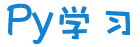注册    登录创作新主题

Python
 python开源   Django   Python   DjangoApp   pycharm
DATA
 docker   Elasticsearch

 问与答   闲聊   招聘   翻译   创业   分享发现   分享创造   求职   区块链   支付之战
aigc
 aigc   chatgpt
WEB开发
 linux   MongoDB   Redis   DATABASE   NGINX   其他Web框架   web工具   zookeeper   tornado   NoSql   Bootstrap   js   peewee   Git   bottle   IE   MQ   Jquery

Python88.com
 反馈   公告   社区推广

Py学习  »  Python

# 手把手教你学python第四课：数据可视化

快速高效学会python的终极奥义：过、抄、仿、改、调、看、练、创、悟

01

## matplotlib介绍

### 基本图形展现

%matplotlib inlineimport matplotlib.pyplot as pltplt.plot([1,3,2,4]，[1,3,2,7

],'ro')plt.ylabel('some numbers')plt.axis([0, 6, 0, 20])plt.show()

axis()命令给定了坐标范围，格式是[xmin, xmax, ymin, ymax]；

# evenly sampled time at 200ms intervalst = np.arange(0., 5., 0.2)# red dashes, blue squares and green trianglesplt.plot(t, t, 'r--', t, t**2, 'bs', t, t**3, 'g^')plt.show()

### 格式设置

#### 线形设置

·我们可以通过'linewidth'来设置线宽，通过设定set_antialiased来确定是否消除锯齿

line = plt.plot([1,2,3,4], [1,4,9,16], '-', linewidth=2.0)line.set_antialiased(False) # 关闭反锯齿

lines = plt.plot([1,2,3,4], [1,4,9,16], [1,2,3,4], [16,5,6,2])# use keyword argsplt.setp(lines, color='r', linewidth=2.0)# or MATLAB style string value pairsplt.setp(lines, 'color', 'r', 'linewidth', 2.0)

线型  描述'-' 实线'--'    虚线'-.'    点划线':' 点'None', '', 不画

标记  描述'o' 圆圈'D' 钻石'h' 六边形1'H' 六边形2'x' X'', 'None'  不画'8' 八边形'p' 五角星',' 像素点'+' 加号'.' 点's' 正方形'*' 星型'd' 窄钻石'v' 下三角''>' 右三角'^' 上三角

标记  颜色'b' 蓝色'g' 绿色'r' 红色'c' 蓝绿色'm' 品红'y' 黄色'k' 黑色'w' 白色

color可以被用在一系列和函数中，这里我们再title中改变color

#### 修改坐标范围

xlim(-2.5, 2.5)#设置y轴范围ylim(-1, 1)plt.plot(x, y1)

#### 创建子图

def f(t):    return np.exp(-t) * np.cos(2*np.pi*t)t1 = np.arange(0.0, 5.0, 0.1)t2 =

np.arange(0.0, 5.0, 0.02)plt.figure(1)plt.subplot(211)plt.plot(t1, f(t1), 'bo', t2, f(t2), 'k')plt.subplot(212)plt.plot(t2, np.cos(2*np.pi*t2), 'r--')plt.show()

plt.figure(1)                # 第一张图plt.subplot(211)             # 第一张图中的第一张子图plt.plot([1,2,3])plt.subplot(212)             # 第一张图中的第二张子图plt.plot([4,5,6])plt.figure(2)                # 第二张图plt.plot([4,5,6])            # 默认创建子图subplot(111)plt.figure(1)                # 切换到figure 1 ; 子图subplot(212)仍旧是当前图plt.subplot(211)             # 令子图subplot(211)成为figure1的当前图plt.title('Easy as 1,2,3')   # 添加subplot 211 的标题

#### 添加文字

text()可以在图中的任意位置添加文字，并支持LaTex语法
xlable(), ylable()用于添加x轴和y轴标签
title()用于添加图的题目

mu, sigma = 100, 15x = mu + sigma * np.random.randn(10000)# 数据的直方图n, bins, patches = plt.hist(x, 50, normed=1, facecolor='g', alpha=0.75)plt.xlabel('Smarts')plt.ylabel('Probability')#添加标题plt.title('Histogram of IQ')#添加文字plt.text(60, .025, r'$\mu=100,\ \sigma=15$')plt.axis([40,

160, 0, 0.03])plt.grid(True)plt.show()t0 = plt.text(0.35,0.5,'my text')plt.setp(t0, color='b',fontsize=24)t = plt.xlabel('my data', fontsize=14, color='red')

#### 注释文本

ax = plt.subplot(111)t = np.arange(0.0, 5.0, 0.01)s = np.cos(2*np.pi*t)line, = plt.plot(t, s, lw=2)plt.annotate('local max', xy=(2, 1), xytext=(3, 1.5),            arrowprops=dict(facecolor='black', shrink=0.05),            )plt.ylim(-2,2)plt.show()

#### 图例

line_up, = plt.plot([1,2,3], label='Line 2')line_down, = plt.plot([3,2,1], label='Line 1')plt.legend(handles=[line_up, line_down])

line1, = plt.plot([1,2,3], label="Line 1", linestyle='--')line2, = plt.plot([3,2,1], label="Line 2", linewidth=4)# Create a legend for the first line.first_legend = plt.legend(handles=[line1], loc=1)# Add the legend manually to the current Axes.ax = plt.gca().add_artist(first_legend)# Create another legend for the second line.plt.legend(handles=[line2], loc=4)plt.show()

## seaborn介绍

### 格式设置 Style management

seaborn有五种模版darkgrid, whitegrid, dark, white, and ticks可以选择

sns.set_style("whitegrid")data = np.random.normal(size=(20, 6)) + np.arange(6) / 2sns.boxplot(data=data);

sns.despine()

you can pass a dictionary of parameters to the rc argument of axes_style() and set_style()

sns.set_style("darkgrid", {"axes.facecolor": ".9"})sinplot()

渐变蓝色：sns.palplot(sns.color_palette("Blues"))渐变绿色：sns.palplot(sns.color_palette("BuGn_r"))渐变紫色：sns.palplot(sns.cubehelix_palette(8))

x, y = np.random.multivariate_normal([0, 0], [[1, -.5], [-.5, 1]], size=300).Tcmap = sns.cubehelix_palette(light=1, as_cmap=True)sns.kdeplot(x, y, cmap=cmap, shade=True);

f, ax = plt.subplots(figsize=(7, 3))sns.countplot(y=

"deck", data=titanic, color="c");

### 各类图像

#### 分布图

##### 单变量分布图

sns.distplot(x, bins=20, kde=False, rug=True，hist=False);sns.kdeplot(x, bw=.2, label="bw: 0.2"，shade=True, cut=0)；sns.distplot(x, kde=False, fit=stats.gamma);
##### 两个变量关系图

sns.jointplot(x="x", y="y", data=df);难一点的蜂窝图x, y = np.random.multivariate_normal(mean, cov, 1000).Twith sns.axes_style("white"):    sns.jointplot(x=x, y=y, kind="hex", color="k");以及密度核型图sns.jointplot(

x="x", y="y", data=df, kind="kde");

f, ax = plt.subplots(figsize=(6, 6))sns.kdeplot(df.x, df.y, ax=ax)sns.rugplot(df.x, color="g", ax=ax)sns.rugplot(df.y, vertical=True, ax=ax);

g = sns.jointplot(x="x", y="y", data=df, kind="kde", color="m")g.plot_joint(plt.scatter, c="w", s=30, linewidth=1, marker="+")g.ax_joint.collections.set_alpha(0)g.set_axis_labels("$X$", "$Y$");

pairplot

iris = sns.load_dataset("iris")sns.pairplot(iris);g = sns.PairGrid(iris)g.map_diag(sns.kdeplot)g.map_offdiag(sns.kdeplot, cmap="Blues_d", n_levels=6);

#### 类别变量

sns.stripplot(x="day", y="total_bill", data=tips);sns.swarmplot(x="day", y="total_bill", data=tips);sns.swarmplot(x="total_bill", y="day", hue="time", data=tips);sns.boxplot(x="day", y="total_bill", hue="time", data=tips);sns.violinplot(x="total_bill", y="day", hue="time", data=tips);

sns.violinplot(x="day", y="total_bill", data=tips, inner=None)sns.swarmplot(x="day", y="total_bill", data=tips,

color="w", alpha=.5);

sns.barplot(x="sex", y="survived", hue="class", data=titanic);sns.countplot(x="deck", data=titanic, palette="Greens_d");sns.pointplot(x="sex", y="survived", hue="class", data=titanic);

sns.factorplot(x="day", y="total_bill", hue="smoker",               col="time", data=tips, kind="swarm");

#### 线性关系图

sns.regplot(x="total_bill", y="tip", data=tips);sns.lmplot(x="total_bill",

y="tip", data=tips);

02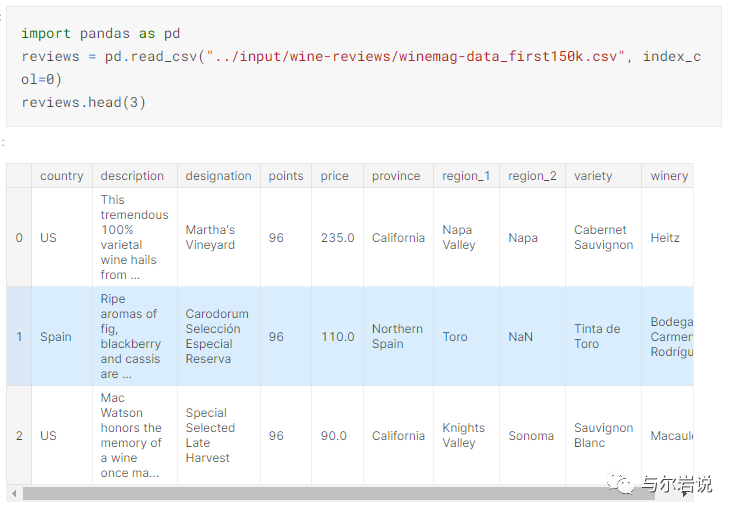## 柱状图

reviews['province'].value_counts().head(10).plot.bar()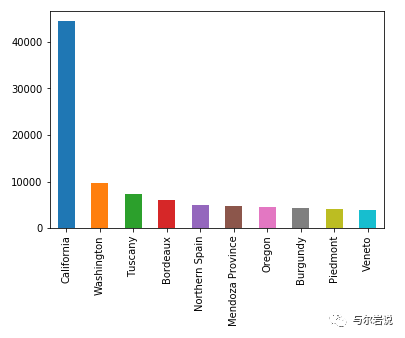(reviews['province'].value_counts().head(10) / len(reviews)).plot.bar()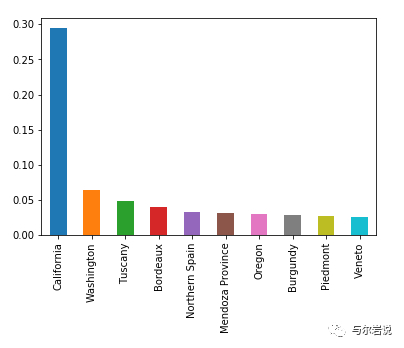reviews['points'].value_counts().sort_index().plot.bar()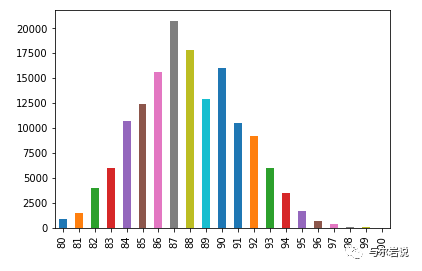## 线图与面积图



reviews['points'].value_counts().sort_index().plot.line()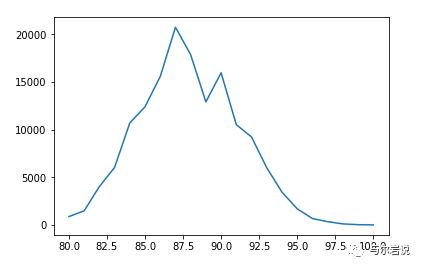reviews['points'].value_counts().sort_index().plot.area()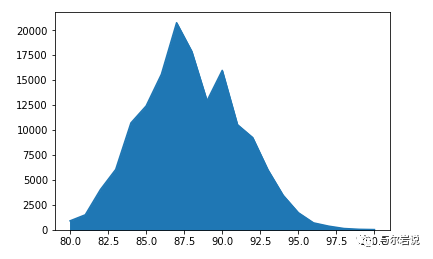## 直方图

reviews[reviews['price'] < 200]['price'].plot.hist()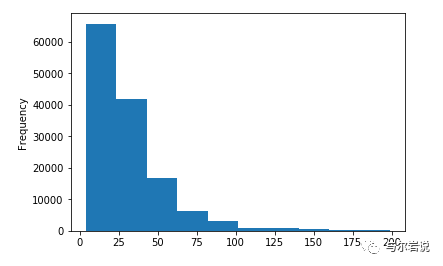## 点状图

reviews[reviews['price'] < 100].sample(100).plot.scatter(x='price', y='points')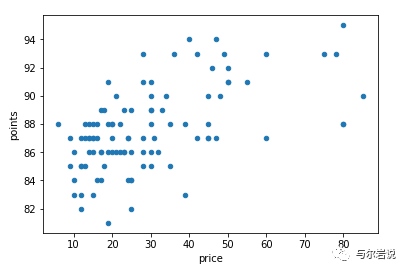reviews[reviews['price'] < 100].plot.scatter(x='price', y='points')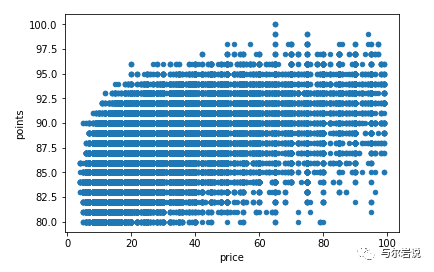## Hexplot图

reviews[reviews['price'] < 100].plot.hexbin(x='price', y='points', gridsize=15)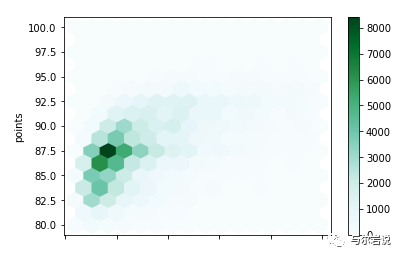## 堆积图

wine_counts.plot.bar(stacked=True)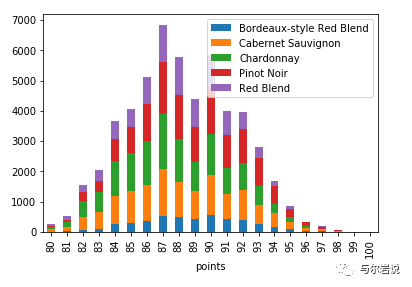wine_counts.plot.area()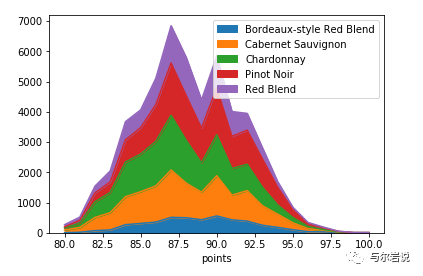## 处理图的格式

import matplotlib.pyplot as pltimport seaborn as snsax = reviews['points'].value_counts().sort_index().plot.bar(    figsize=(12, 6),    color='mediumvioletred',    fontsize=16)ax.set_title("Rankings Given by Wine Magazine", fontsize=20)sns.despine(bottom=True, left=True)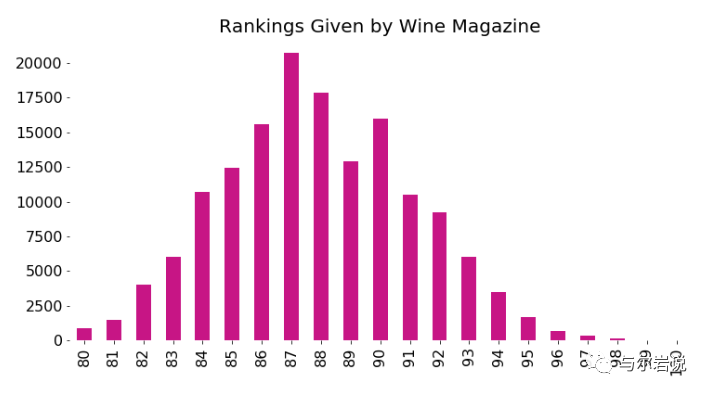03

## Countplot

sns.countplot(reviews['points'])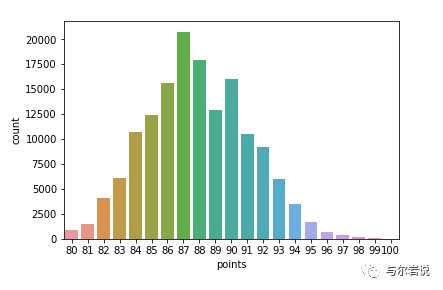seaborn不需要我们通过数值计算为它塑造数据；countplot为我们聚合数据。

## KDE plot

KDE是一种消除数据噪声的统计方法。它解决了折线图的一个重要的基本弱点：它会去除异常值或“中间值”，这将导致折线图突然下降。

KDE图比折线图更能获得区间数据的“真实形状”。事实上，对于这样的数据，我建议总是使用它而不是折线图。

sns.kdeplot(reviews.query('price < 200').price)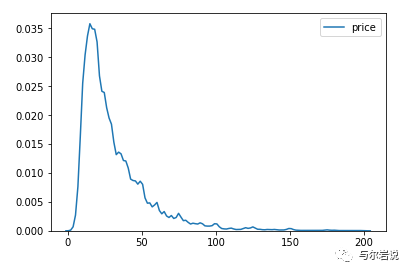KDE图也可以用于二维。

sns.kdeplot(reviews[reviews['price'] < 200].loc[:, ['price', 'points']].dropna().sample(5000))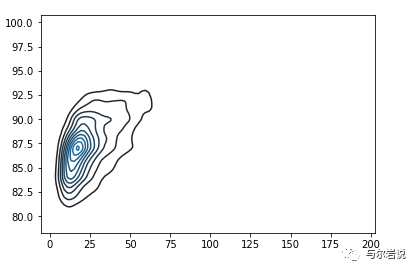## Distplot

sns.distplot(reviews['points'], bins=10, kde=False)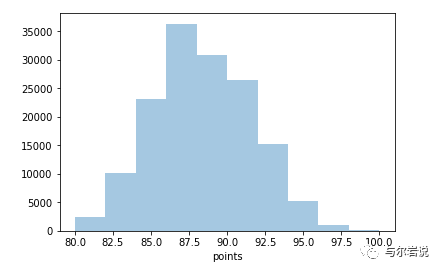sns.jointplot(x='price', y='points', data=reviews[reviews['price'] < 100])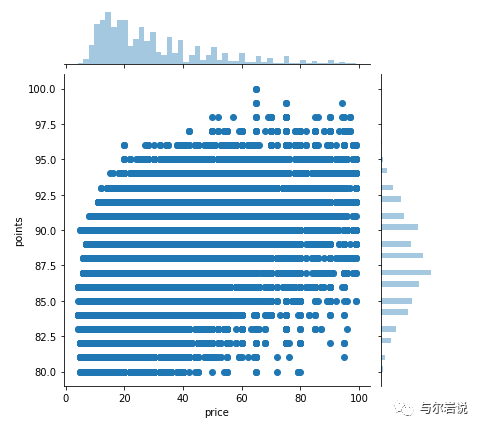Notice that this plot comes with some bells and whistles: a correlation coefficient is provided, along with histograms on the sides. These kinds of composite plots are a recurring theme in seaborn. Other than that, the jointplot is just like the pandas scatter plot.

As in pandas, we can use a hex plot (by simply passing kind='hex') to deal with overplotting:

sns.jointplot(x='price', y='points', data=reviews[reviews['price'] < 100], kind='hex',               gridsize=20)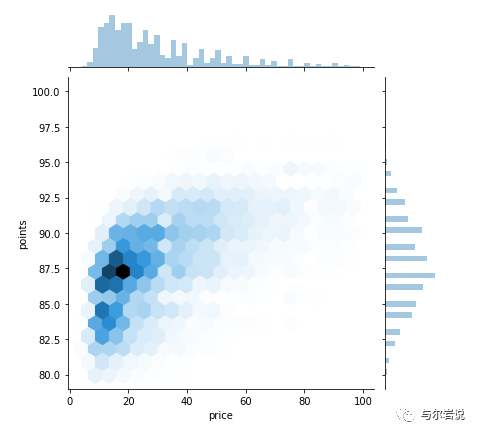## Boxplot

df = reviews[reviews.variety.isin(reviews.variety.value_counts().head(5).index)]sns.boxplot(    x='variety',    y='points',    data=df)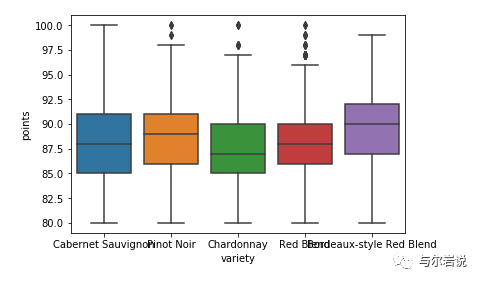sns.violinplot(    x='variety',    y='points',    data=reviews[reviews.variety.isin(reviews.variety.value_counts()[:5].index)])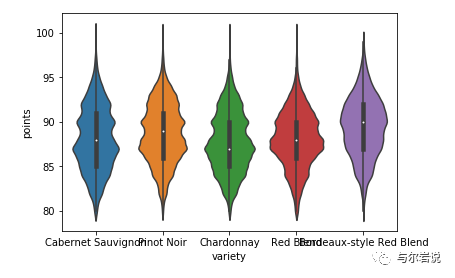## Subplot分图

fig, axarr = plt.subplots(2, 2, figsize=(12, 8))reviews['points'].value_counts().sort_index().plot.bar(    ax=axarr, fontsize=12, color='mediumvioletred')axarr.set_title("Wine Scores", fontsize=18)reviews['variety'].value_counts().head(20).plot.bar(    ax=axarr, fontsize=12, color='mediumvioletred')axarr.set_title("Wine Varieties", fontsize=18)reviews['province'].value_counts().head(20).plot.bar(    ax=axarr, fontsize=12, color='mediumvioletred')axarr.set_title("Wine Origins", fontsize=18)reviews['price'].value_counts().plot.hist(    ax=axarr, fontsize=12, color='mediumvioletred')axarr.set_title("Wine Prices", fontsize=18)plt.subplots_adjust(hspace=.3)import seaborn as snssns.despine()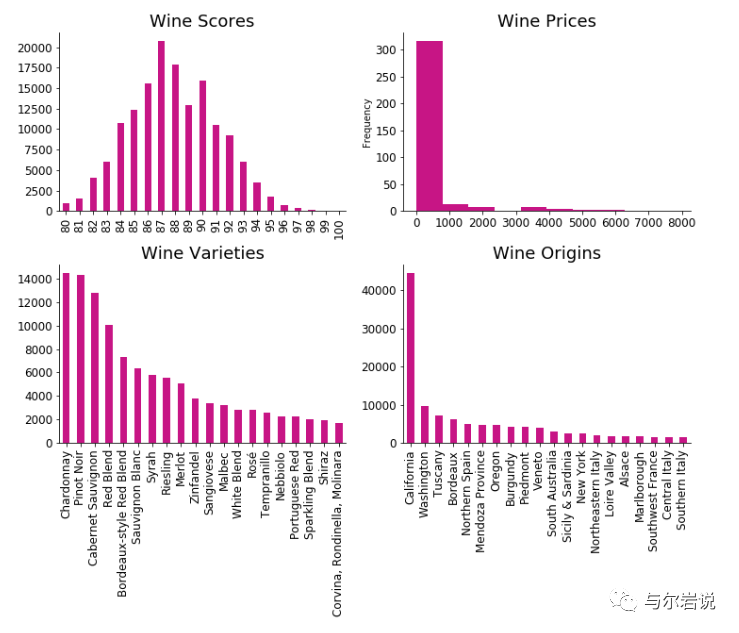## Facet刻面

df = footballers[footballers['Position'].isin(['ST', 'GK'])]df = df[df['Club'].isin(['Real Madrid CF', 'FC Barcelona', 'Atlético Madrid'])]g = sns.FacetGrid(df, row="Position", col="Club")g.map(sns.violinplot, "Overall")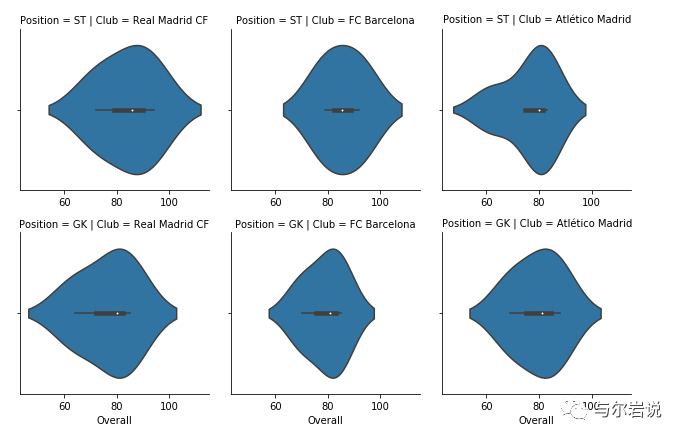pairplot是一种非常有用且广泛使用的seaborn方法，用于刻面变量（与变量值相反）。将正确形状的数据帧传递给它，它将返回变量值的网格化结果：

sns.pairplot(footballers[['Overall', 'Potential', 'Value']])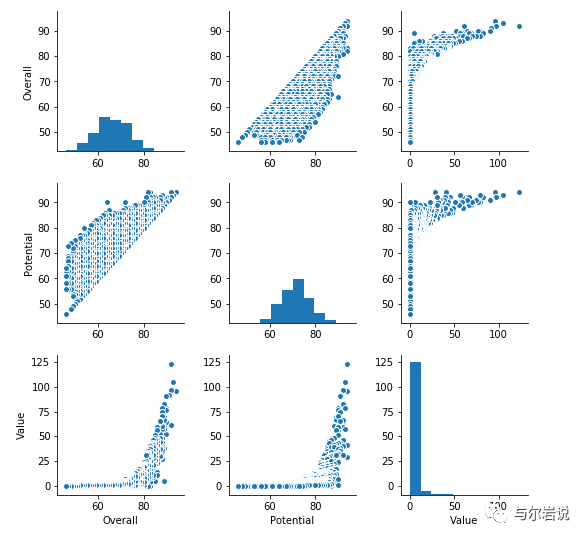## lmplot

import seaborn as snssns.lmplot(x='Attack', y='Defense', hue='Legendary',            markers=['x', 'o'],           fit_reg=False, data=pokemon)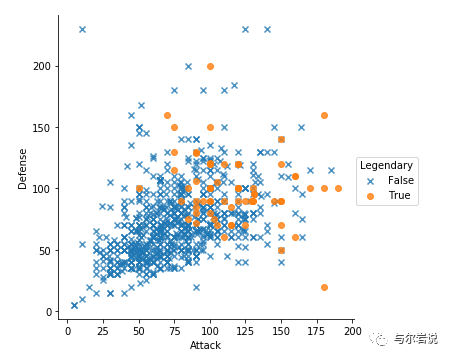## heatmap plot相关系数

sns.heatmap(    pokemon.loc[:, ['HP', 'Attack', 'Sp. Atk', 'Defense', 'Sp. Def', 'Speed']].corr(),    annot=True)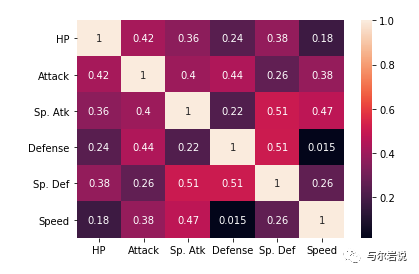## parallel_coordinates

import pandas as pdfrom pandas.plotting import parallel_coordinatesp = (pokemon[(pokemon['Type 1'].isin(["Psychic", "Fighting"]))]         .loc[:, ['Type 1', 'Attack', 'Sp. Atk', 'Defense', 'Sp. Def']]    )parallel_coordinates(p, 'Type 1')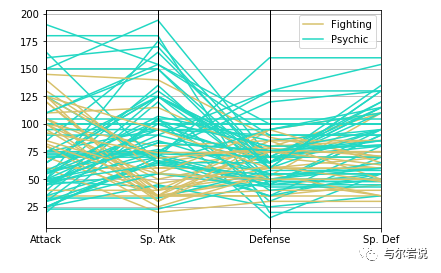04

matplotlib画状态切分图～～之前也有很多小伙伴感兴趣

fig,ax1=plt.subplots(figsize=(20,7)) plt.plot(data['剩余流动性因子'],'black',label='剩余流动性因子') 

plt.legend(loc='lower left') ax1.set_ylabel('剩余流动性因子') ax2=ax1.twinx() plt.plot(data['通胀因子'],label='通胀因子') ax2.set_ylabel('通胀因子') plt.legend() dd=dd.dropna() con1=0 con2=0 con3=0 con4=0 for i in dd.index:     if dd.loc[i,'剩余流动性因子_上行概率']<0.5 and dd.loc[i,'通胀因子_上行概率']<0.5:         num=list(dd.index).index(i)+1         plt.axvspan(i,list(dd.index)[num],label="_"*con1+"通胀下行剩余流动性下行",alpha=0.3)         plt.legend()         con1=con1+1     if dd.loc[i,'剩余流动性因子_上行概率']>0.5 and dd.loc[i,'通胀因子_上行概率']<0.5:         num=list(dd.index).index(i)+1         plt.axvspan(i,list(dd.index)[num],label="_"*con2+"通胀下行剩余流动性上行",color='darkcyan',alpha=0.3)         plt.legend()         con2=con2+1     if dd.loc[i,'剩余流动性因子_上行概率']<0.5 and dd.loc[i,'通胀因子_上行概率']>0.5:         try:             num=list(dd.index).index(i)+1             plt.axvspan(i,list(dd.index)[num],label="_"*con3+"通胀上行剩余流动性下行",color='moccasin',alpha=0.3)         except:             print(i)         con3=con3+1     if dd.loc[i,'剩余流动性因子_上行概率']>0.5 and dd.loc[i,'通胀因子_上行概率']>0.5:         try:             num=list(dd.index).index(i)+1             plt.axvspan(i,list(dd.index)[num],label="_"*con4+"通胀上行剩余流动性上行",color='orange',alpha=0.3)             con4=con4+1         except:             print(i) plt.legend()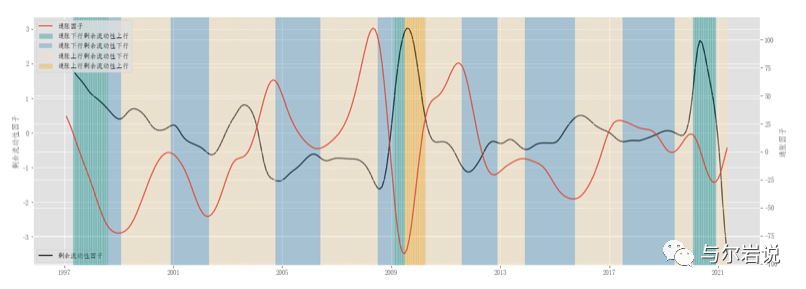from plotly.offline import init_notebook_mode, iplotinit_notebook_mode(connected=True)

import plotly.graph_objs as goiplot([go.Scatter(x=reviews.head(1000)['points'], y=reviews.head(1000)['price'], mode='markers')])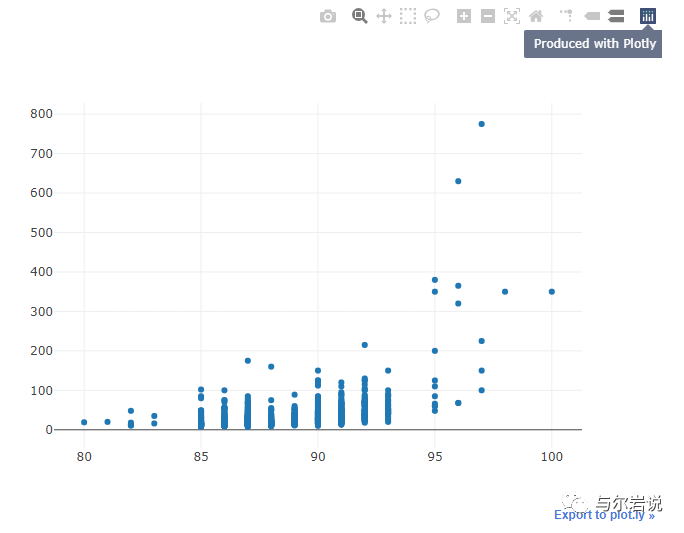iplot([go.Histogram2dContour(x=reviews.head(500)['points'],                              y=reviews.head(500)['price'], 

contours=go.Contours(coloring='heatmap')),       go.Scatter(x=reviews.head(1000)['points'], y=reviews.head(1000)['price'], mode='markers')])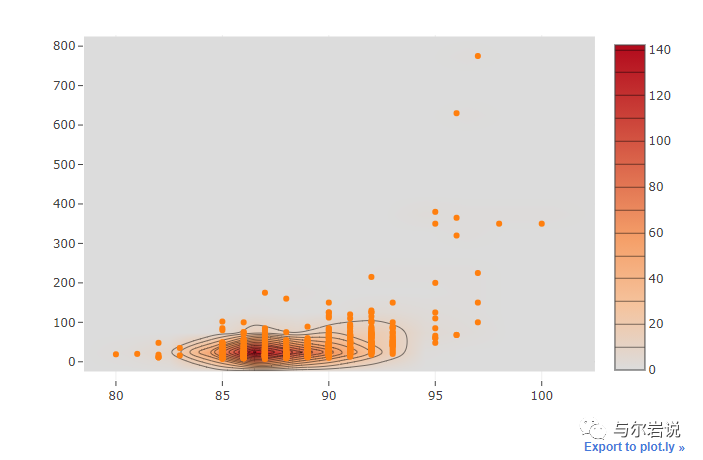plotly公开了几种不同的API，其复杂性从低级到高级不等。iplot是最高级别的API，因此是最方便的通用API。

df = reviews.assign(n=0).groupby(['points', 'price'])['n'].count().reset_index()df = df[df["price"] < 100]v = df.pivot(index='price', columns='points', values='n').fillna(0).values.tolist()iplot([go.Surface(z=v)])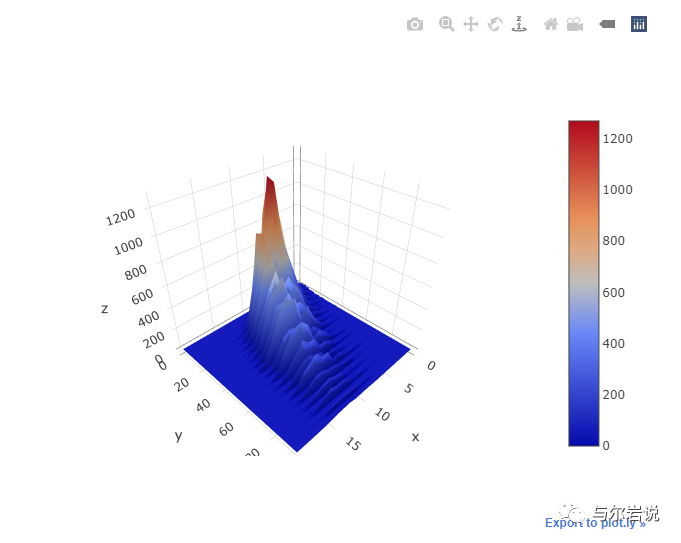df = reviews['country'].replace("US", "United States").value_counts()iplot([go.Choropleth(    locationmode='country names',    locations=df.index.values,    text=df.index,    z=df.values)])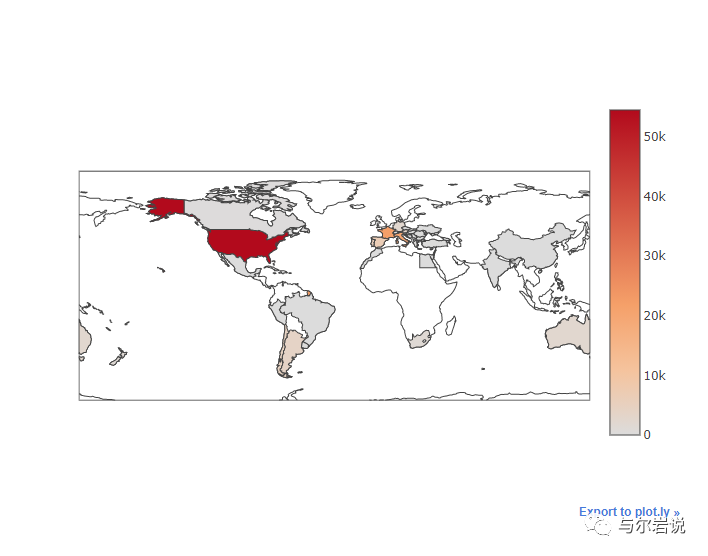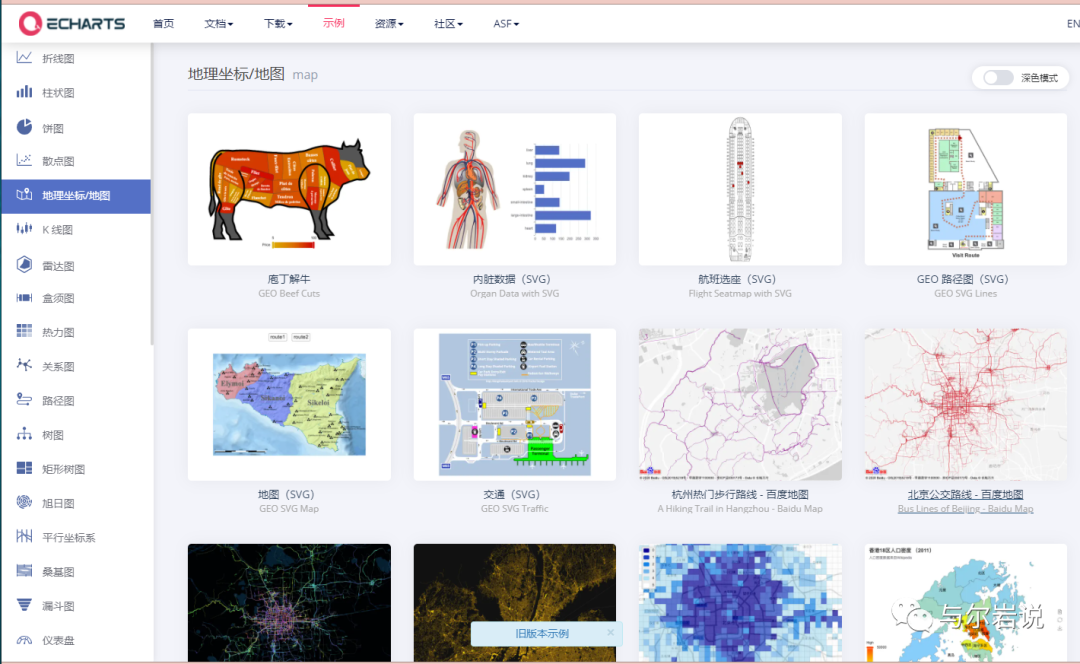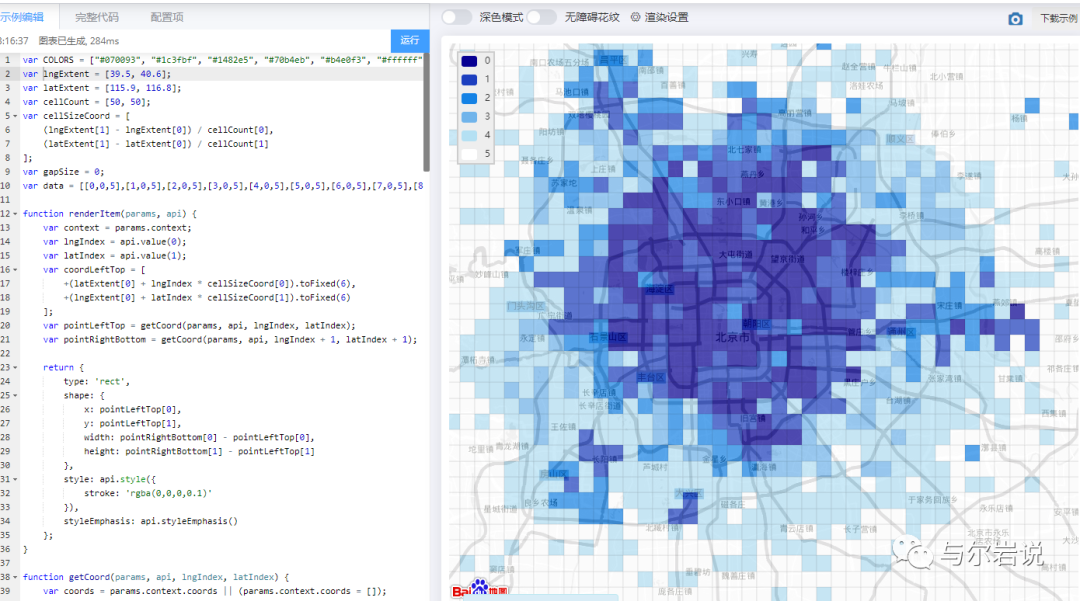Python社区是高质量的Python/Django开发社区